# Beta regression for the microbiome data

### Chapter 4.3.3: Generalized linear models

This model regresses a measure of microbiome species richness onto features of the home from which the sample was taken. Let $$OTU_{ij}$$ be the abundance of Operational Taxonomic Unit (OTU) $$j$$ in sample $$i$$. The response variable is the proportion of the abundance attributed to the most abundant OTU, $Y_i = \frac{\mbox{max}\{OTU_{i1},…,OTU_{im}\}}{\sum_{j}OTU_{ij}}\in(0,1).$ There are eight covariates ($$X_{ij}$$):

1. Longitude
2. Latitude
3. Annual average temperature
4. Annual average precipitation
5. Net primary production
6. Elevation
7. The binary indicator of whether it is a single-family home
8. Number of bedrooms

The regression model is $Y_i\sim\mbox{Beta}(rq_i,r(1-q_i)) \mbox{ where logit}(q_i) = \sum_{l=1}^pX_{ij}\beta_j,$ so that the expected value of $$Y_i$$ is $$q_i\in[0,1]$$ and the concentration around $$q_i$$ is determined by $$r>0$$. The regression coefficients have uninformative priors $$\beta_j\sim\mbox{Normal}(0,10^2)$$ and the concentration parameter has prior $$r\sim\mbox{Gamma}(0.1,0.1)$$.

## Load the data

 set.seed(0820)

load("S:\\Documents\\My Papers\\BayesBook\\Data\\Microbiome\\homes.RData")
ls()

##  "homes" "OTU"

 city     <- homes[,2]
state    <- homes[,3]
lat      <- homes[,4]
long     <- homes[,5]
temp     <- homes[,6]
precip   <- homes[,7]
NPP      <- homes[,8]
elev     <- homes[,9]
house    <- ifelse(homes[,10]=="One-family house detached from any other house",1,0)
bedrooms <- as.numeric(homes[,11])

## Warning: NAs introduced by coercion

 OTU      <- as.matrix(OTU)
Y        <- apply(OTU,1,max)/rowSums(OTU)
X        <- cbind(long,lat,temp,precip,NPP,elev,house,bedrooms)
names    <- c("Intercept","Longitude","Latitude",
"Temperature","Precipitation","NPP",
"Elevation","Single-family home",
"Number of bedrooms")

# Remove observations with missing values
junk        <- is.na(rowSums(X))
Y           <- Y[!junk]
X           <- X[!junk,]
city        <- city[!junk]
state       <- state[!junk]

# Standardize the covariates
X           <- as.matrix(scale(X))

X           <- cbind(1,X) # add the intercept
colnames(X) <- names
n           <- length(Y)
p           <- ncol(X)


## Plot the data

hist(Y,breaks=50,ylab="Maximum proportion")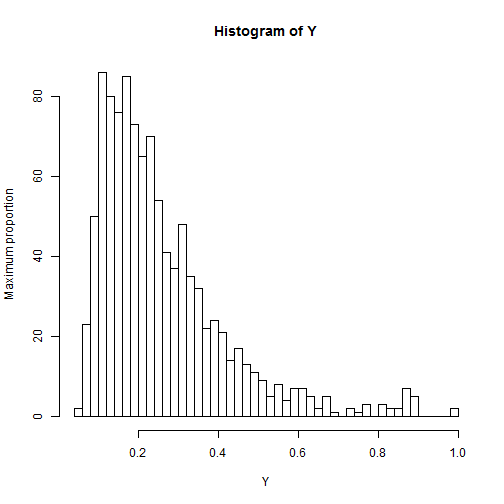for(j in 2:p){
plot(X[,j],Y,xlab=names[j],ylab="Maximum proportion")
}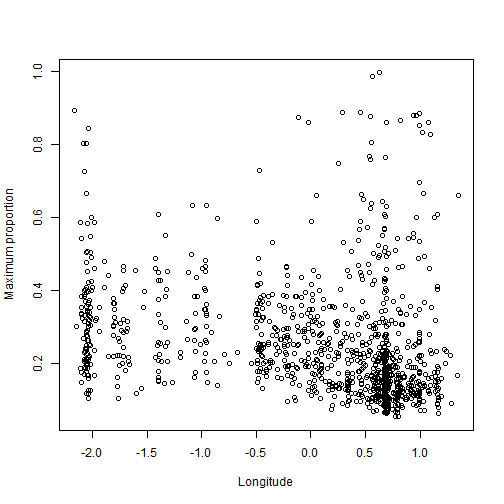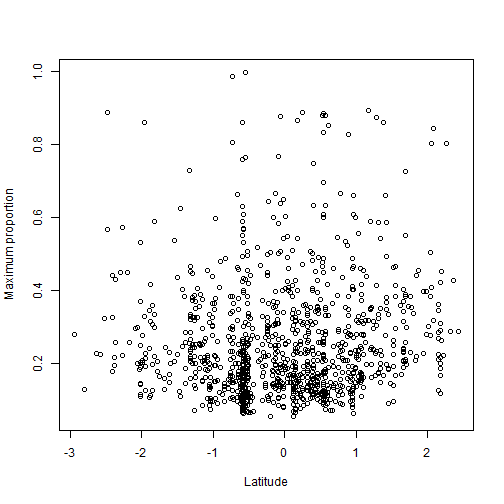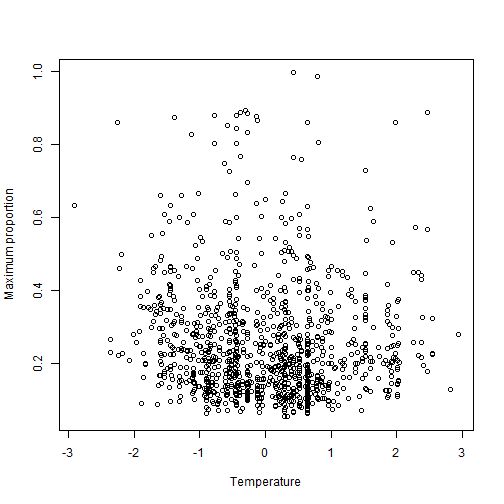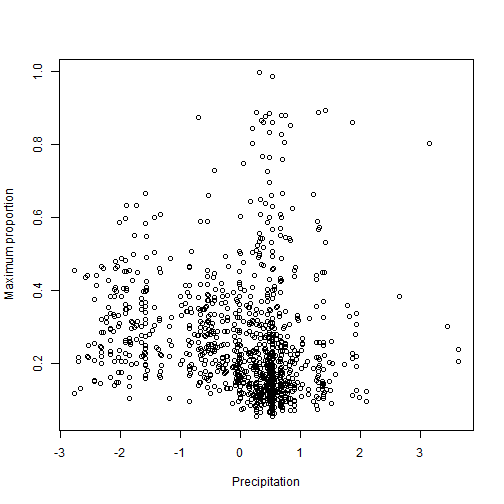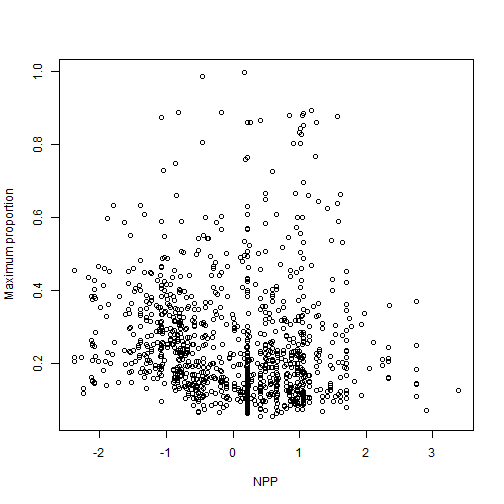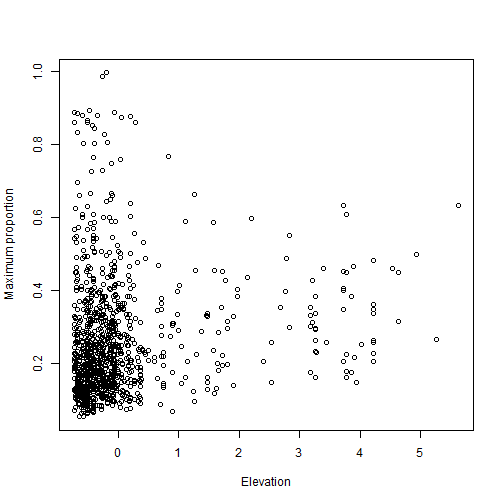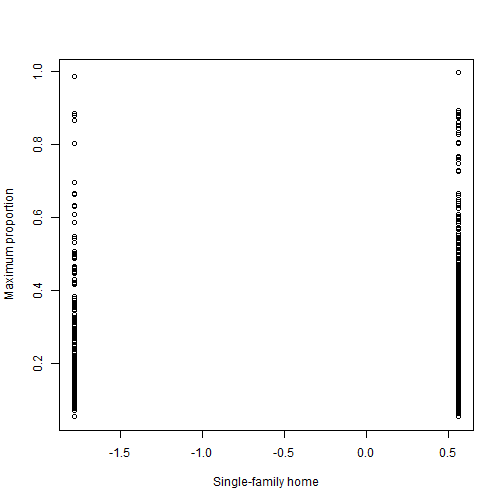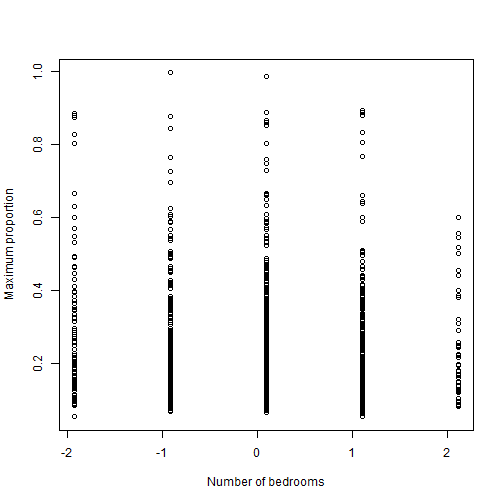## Fit the beta regression model in JAGS

 library(rjags)

data   <- list(Y=Y,X=X,n=n,p=p)
params <- c("beta","r")

model_string <- textConnection("model{
for(i in 1:n){
Y[i]       ~ dbeta(r*mu[i],r*(1-mu[i]))
logit(mu[i]) <- inprod(X[i,],beta[])
}
for(j in 1:p){beta[j] ~ dnorm(0,0.01)}
r ~ dgamma(0.1,0.1)
}")

model <- jags.model(model_string,data = data, n.chains=2,quiet=TRUE)
update(model, 10000, progress.bar="none")
samples <- coda.samples(model, variable.names=params, thin=5, n.iter=20000, progress.bar="none")

plot(samples)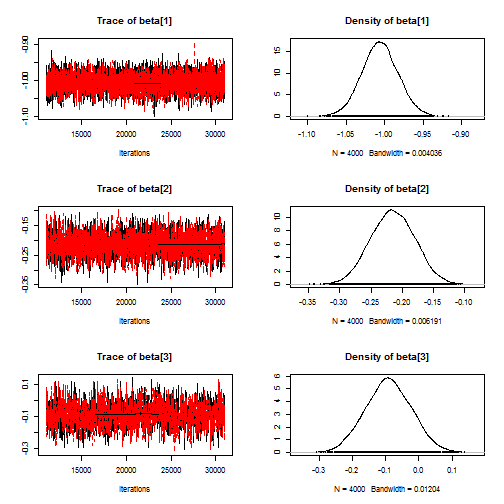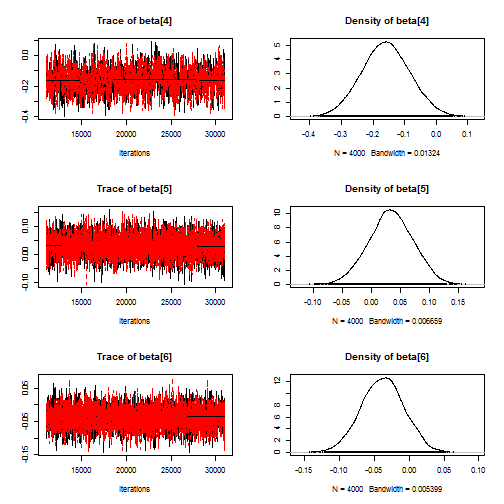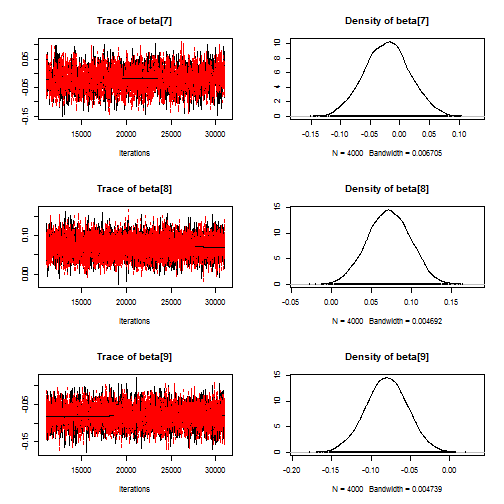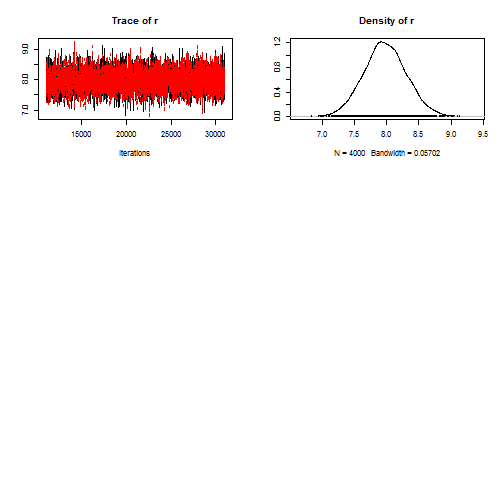sum <- summary(samples)

rownames(sum$statistics) <- c(names,"r") rownames(sum$quantiles)  <- c(names,"r")
sum$statistics <- round(sum$statistics,3)
sum$quantiles <- round(sum$quantiles,3)
sum

##
## Iterations = 11005:31000
## Thinning interval = 5
## Number of chains = 2
## Sample size per chain = 4000
##
## 1. Empirical mean and standard deviation for each variable,
##    plus standard error of the mean:
##
##                      Mean    SD Naive SE Time-series SE
## Intercept          -1.006 0.023    0.000          0.000
## Longitude          -0.215 0.035    0.000          0.001
## Latitude           -0.091 0.069    0.001          0.002
## Temperature        -0.159 0.075    0.001          0.002
## Precipitation       0.033 0.038    0.000          0.001
## NPP                -0.038 0.031    0.000          0.000
## Elevation          -0.021 0.038    0.000          0.001
## Single-family home  0.072 0.027    0.000          0.000
## Number of bedrooms -0.080 0.027    0.000          0.000
## r                   7.977 0.330    0.004          0.004
##
## 2. Quantiles for each variable:
##
##                      2.5%    25%    50%    75%  97.5%
## Intercept          -1.052 -1.022 -1.006 -0.991 -0.960
## Longitude          -0.284 -0.239 -0.215 -0.191 -0.146
## Latitude           -0.224 -0.138 -0.091 -0.044  0.044
## Temperature        -0.306 -0.211 -0.160 -0.109 -0.010
## Precipitation      -0.043  0.008  0.033  0.059  0.106
## NPP                -0.098 -0.059 -0.038 -0.017  0.020
## Elevation          -0.097 -0.047 -0.021  0.004  0.055
## Single-family home  0.020  0.054  0.072  0.090  0.123
## Number of bedrooms -0.134 -0.098 -0.080 -0.062 -0.027
## r                   7.336  7.758  7.972  8.193  8.638